### MINUTE Function Examples – Excel, VBA, & Google Sheets

This tutorial demonstrates how to use the Excel MINUTE Function in Excel to get the minute of time.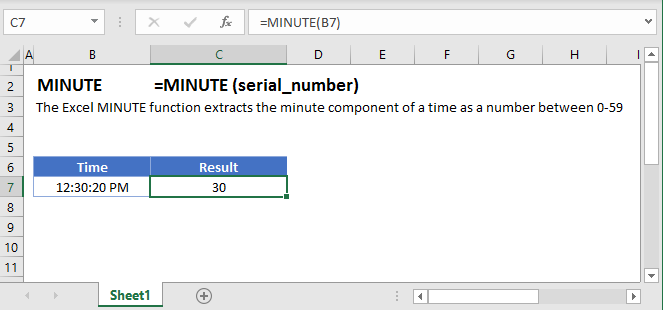## MINUTE Function Overview

The MINUTE Function Returns the minute as a number (0-59).

To use the MINUTE Excel Worksheet Function, Select cell and Type: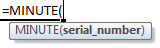(Notice how the formula input appear)

## MINUTE Function syntax and Inputs:

serial_number – The time, represented as a fraction of the day or entered as time surrounded by quotations (“s”). Example: You can not enter 8:00:00 pm directly into the cell. Instead you would need to use the corresponding fraction of the day: .3333 or the time surrounded by quorations: “8:00:00 pm”. Alternatively, you can reference a cell with the time entered. Excel automatically converts times stored in cells into a fractional number (unless the time is stored as text).

AutoMacro - VBA Code Generator

## Minute Examples

The MINUTE Function returns the minute number of a time: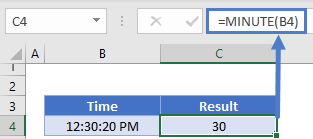### MINUTE and TIME Functions

Using the TIME and MINUTE Functions you can create a new time with the minute number from the original time: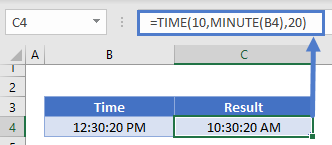## MINUTE in Google Sheets

The MINUTE Function works exactly the same in Google Sheets as in Excel: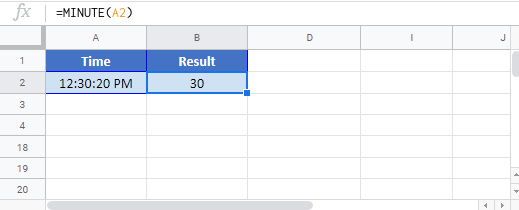## MINUTE Examples in VBA

You can also use the MINUTE function in VBA. Type:
`application.worksheetfunction.minute(serial_number)`
For the function arguments (serial_number, etc.), you can either enter them directly into the function, or define variables to use instead.

Return to the List of all Functions in Excel

## Excel Practice Worksheet

Practice Excel functions and formulas with our 100% free practice worksheets!

• Automatically Graded Exercises
• Learn Excel, Inside Excel!